This article summarizes the findings of a paper entitled, “Prediction of Spot Weld Failure in Automotive Steels,”L-48 authored by J. H. Lim and J.W. Ha, POSCO, as presented at the 12th European LS-DYNA Conference, Koblenz, 2019.

To better predict car crashworthiness it is important to have an accurate prediction of spot weld failure. A new approach for prediction of resistance spot weld failure was proposed by POSCO researchers. This model considers the interaction of normal and bending components and calculating the stress by dividing the load by the area of plug fracture.

## Background

Lee, et al.L-49 developed a model to predict spot welding failure under combined loading conditions using the following equation, based upon experimental results .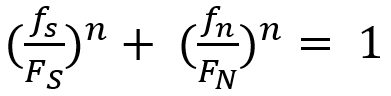Equation 1

Where FS and FN are shear and normal failure load, respectively, and n is a shape parameter.

Later, Wung and coworkersW-38 developed a model to predict the failure mode based upon the normal load, shear load, bending and torsion as shown in Equation 2.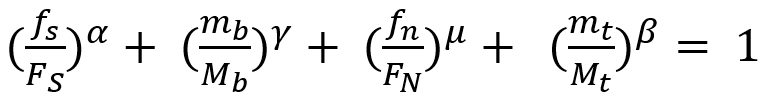Equation 2

Here, FS, FN ,Mb and Mt are normal failure load, shear failure load, failure moment and failure torsion of spot weld, respectively.  α, β, γ and μ are shape parameters.

Seeger et al.S-106 proposed a model for failure criterion that describes a 3D polynomial failure surface. Spot weld failure occurs if the sum of the components of the normal, bending and shear stresses are above the surface, as shown in the Figure 1.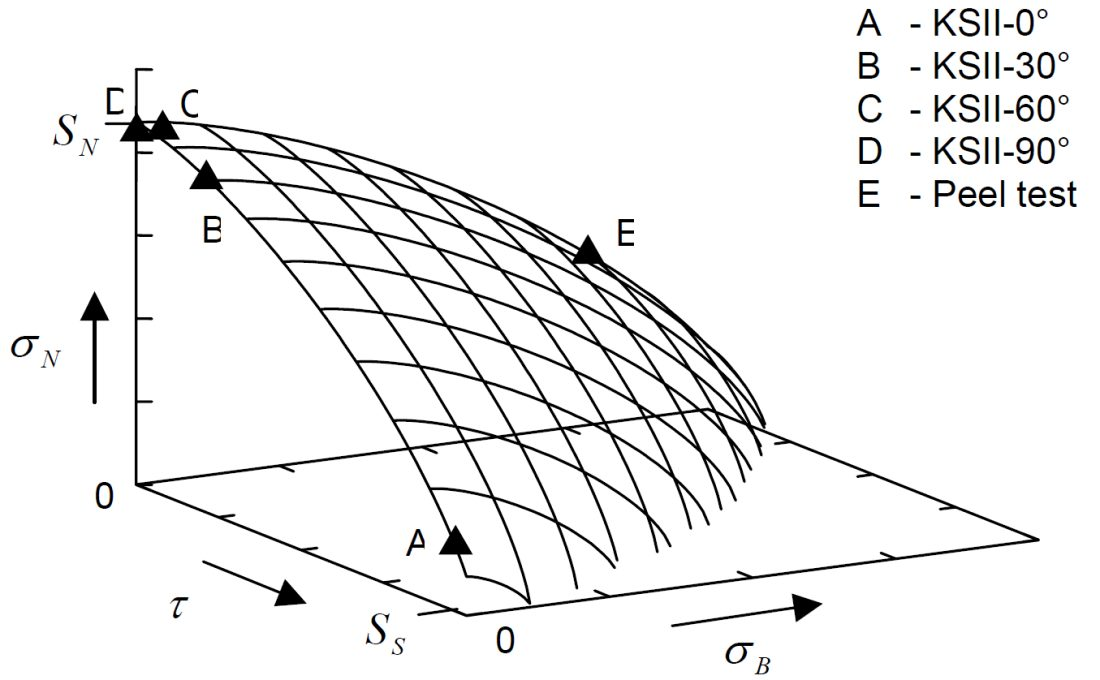Figure 1:  Spot weld failure model proposed by Seeger et al.S-106

The failure criterion can be expressed via Equation 3.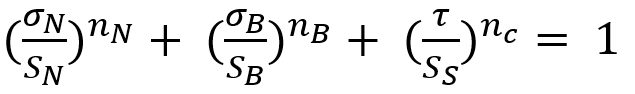Equation 3

Here, σN , σB , and τ are normal, bending and shear stress of the spot weld, respectively. And nN, nB and nc are the shape parameters. Toyota Motor CorporationL-50 has developed the stress-based failure model as shown in Equation 4.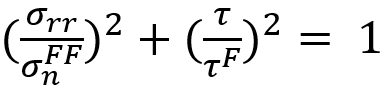Equation 4

## Hybrid Method to Determine Coefficients for Failure Models

This work used a unique hybrid method to determine the failure coefficients for modeling. The hybrid procedure steps are as follows:

2. Finite element simulations are performed for each experiment.
3. Based on the failure loads obtained in each test, the instant of onset of spot weld failure is determined. Failure loads are extracted comparing experiments with simulations.
4. Post processing of those simulations gives the failure load components acting on spot welds such as normal, shear and bending loads.

These failure load components are plotted on the plane consisting of normal, shear and bending axes.

The hybrid method described above is shown in Figure 2.L-48Figure 2:  Hybrid method to obtain the failure load with respect to test conditions.L-48

## New Spot Weld Failure Model

The new proposed spot weld failure model in this paper considers only plug fracture mode as a normal spot weld failure. Secondly normal and bending components considered to be dependent upon each other. Stress generated by normal and bending components is shear, and shear component generates normal stress. Lastly authors have used πdt to calculate the area of stress instead of πd2/4. The final expression is shown in the Equation 5.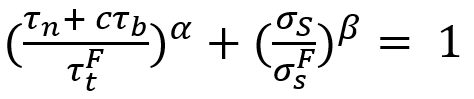Equation 5

Here τn is the shear stress by normal load components, σS is the normal stress due to shear load component. And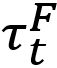,, c, α and β are coefficients.

This work included verification experiments of 42 kinds of homogenous steel stack-ups and 23 heterogeneous stack-ups. The strength levels of the steels used was between 270 MPa and 1500 MPa, and thickness between 0.55 mm and 2.3 mm. These experiments were used to evaluate the model and compare the results to the Wung model.

## Conclusions

Overall, this new model considers interaction between normal and bending components as they have the same loading direction and plane. The current developed model was compared with the Wung model described above and has shown better results with a desirable error, especially for asymmetric material and thickness.

Related Posts
Modern car bodies today are made of increasing volumes of Advanced High-Strength Steels (AHSS), the superb

18

This article summarizes a paper entitled, “RSW of 22MnB5 at Overlaps with Gaps-Effects, Causes, and

18

18

The advantages of numerical simulations for

18

Judging Weldability Using Carbon Equivalence (CE) Existing Carbon Equivalent (CE) formulas for Resistance

18

In general, if any type of AHSS [

18

Recently research at the Ohio State

18

Figure 1 shows a typical cross-section of a

18

Among various dissimilar material combinations, Al-steel is one of the most desirable combination. This section will

18